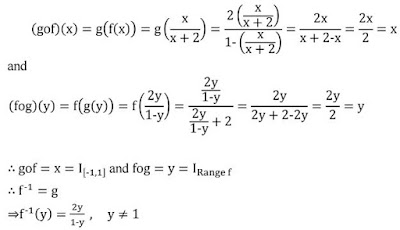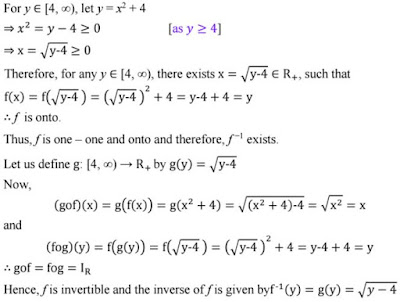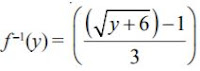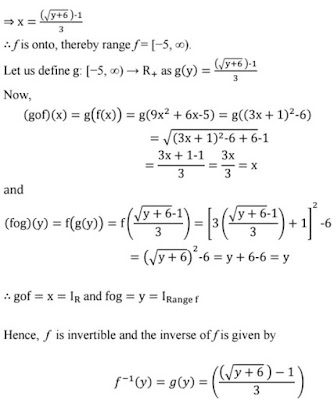#### NCERT Solutions for Class 12th: Ch 1 Relations and Functions Exercise 1.3 Math

Page No: 18

Exercise 1.3

1. Let f : {1, 3, 4} → {1, 2, 5} and g : {1, 2, 5} → {1, 3} be given by f = {(1, 2), (3, 5), (4, 1)} and g = {(1, 3), (2, 3), (5, 1)}. Write down gof

The functions f: {1, 3, 4} → {1, 2, 5} and g: {1, 2, 5} → {1, 3} are defined as f = {(1, 2), (3, 5), (4, 1)} and g = {(1, 3), (2, 3), (5, 1)}.
gof(1) = g[f(1)] = g(2) = 3    [as f(1) = 2 and g(2) = 3]
gof(3) = g[f(3)] = g(5) = 1    [as f(3) = 5 and g(5) = 1]
gof(4) = g[f(4)] = g(1) = 3    [as f(4) = 1 and g(1) = 3]
∴ gof = {(1, 3), (3, 1), (4, 3)}

2. Let f, g and h be functions from R to R. Show that
(f + g) oh = foh + goh
(f . g) oh = (foh) . (goh)

To prove: (f + g)oh = foh + goh
LHS = [(f + g)oh](x)
= (f + g)[h(x)]
= f [h(x)] + g[h(x)]
= (foh)(x) + (goh)(x)
= {(foh)(x) + (goh)}(x) = RHS
∴ {(f + g)oh}(x) = {(foh)(x) + (goh)}(x) for all x ∈R
Hence, (f + g)oh = foh + goh

To Prove: (f.g)oh = (foh).(goh)
LHS = [(f.g)oh](x)
= (f.g)[h(x)]
= f[h(x)] . g[h(x)]
= (foh)(x) . (goh)(x)
= {(foh).(goh)}(x) = RHS
∴ [(f.g)oh](x) = {(foh).(goh)}(x)    for all x ∈R
Hence, (f.g)oh = (foh).(goh)

3. Find gof and fog, if
(i) f(x) = | x | and g(x) = | 5x – 2 |
(ii) f(x) = 8x3 and g(x) = x1/3.

(i). f(x) = |x| and g(x) = |5x-2|
∴gof(x) = g(f(x)) = g(|x|) = |5|x|-2|
fog(x) = f(g(x)) = f(|5x-2|) = ||5x-2|| = |5x-2|

(ii). f(x) = 8x3 and g(x) = x1/3
∴gof(x) = g(f(x)) = g(8x3) = (8x3)1/3 = 2x
fog(x) = f(g(x)) = f(x1/3) = 8(x1/3)3 = 8x

4. If f(x) = (4x+3)/(6x-4), x ≠ 2/3, show that fof(x) = x, for all x ≠ 2/3. What is the inverse of f ?Hence, the given function f is invertible and the inverse of f is f itself.

5. State with reason whether following functions have inverse
(i) f : {1, 2, 3, 4} → {10} with
f = {(1, 10), (2, 10), (3, 10), (4, 10)}

(ii) g : {5, 6, 7, 8} → {1, 2, 3, 4} with
g = {(5, 4), (6, 3), (7, 4), (8, 2)}

(iii) h : {2, 3, 4, 5} → {7, 9, 11, 13} with
h = {(2, 7), (3, 9), (4, 11), (5, 13)}

(i) f: {1, 2, 3, 4} → {10} defined as f = {(1, 10), (2, 10), (3, 10), (4, 10)}
From the given definition of f, we can see that f is a many one function as f(1) = f(2) = f(3) = f(4) = 10
∴f is not one – one.
Hence, function f does not have an inverse.

(ii) g: {5, 6, 7, 8} → {1, 2, 3, 4} defined as g = {(5, 4), (6, 3), (7, 4), (8, 2)}
From the given definition of g, it is seen that g is a many one function as g(5) = g(7) = 4.
∴ g is not one – one.
Hence, function g does not have an inverse.

(iii) h: {2, 3, 4, 5} → {7, 9, 11, 13} defined as h = {(2, 7), (3, 9), (4, 11), (5, 13)}
It is seen that all distinct elements of the set {2, 3, 4, 5} have distinct images under h.
∴ Function h is one – one.
Also, h is onto since for every element y of the set {7, 9, 11, 13}, there exists an element x in the set {2, 3, 4, 5}, such that h(x) = y.
Thus, h is a one – one and onto function.
Hence, h has an inverse.

6. Show that f : [–1, 1] → R, given by f (x) =  x/(x+2)  is one-one. Find the inverse of the function f : [–1, 1] → Range f.
[Hint: For y ∈ Range f, y = f(x) =  x/(x+2), for some x in [–1, 1], i.e., x = 2y/(2y-1)]

f : [–1, 1] → R, given by f (x) =  x/(x+2)
For one-one,Now,7. Consider f : R → R given by f(x) = 4x + 3. Show that f is invertible. Find the inverse of f.

f: R → R is given by, f(x) = 4x + 3
For one – one
Let f(x) = f(y)
⇒ 4x + 3 = 4y + 3
⇒ 4x = 4y
⇒ x = y
∴ f is a one – one function.

For onto
For y ∈ R, let y = 4x + 3.
⇒ x = y-3/4 ∈ R
Therefore, for any y ∈ R, there exists x = y-3/4 ∈ R, such that8. 8. Consider f : R+ → [4, ∞) given by f(x) = x2 + 4. Show that f is invertible with the inverse f-1 of f given by f-1(y) = √(y-4) , where R+ is the set of all non-negative real numbers.

f: R+ → [4, ∞) is given as f(x) = x2 + 4.

For one – one
Let f(x) = f(y)
⇒ x2 + 4 = y2 + 4
⇒ x2 = y2
⇒ x = y [as x = y ∈ R+]
∴ f is a one–one function.

For ontoPage No. 19

9. Consider f : R+ → [– 5, ∞) given by f (x) = 9x2 + 6x - 5. Show that f is invertible withf: R+ → [−5, ∞) is given as f(x) = 9x2 + 6x − 5.
Let y be an arbitrary element of [−5, ∞).
Let y = 9x2 + 6x – 5
⇒ y = (3x+1)2 -1 - 5 = (3x+1)2 -6
⇒ y + 6 = (3x + 1)2
⇒ 3x + 1 = √(y + 6)10. Let f : X → Y be an invertible function. Show that f has unique inverse.
(Hint: suppose g1 and g2 are two inverses of f. Then for all y ∈ Y, fog1(y) = IY(y) = fog2 (y). Use one-one ness of f).

Let f: X → Y be an invertible function.
Also, suppose f has two inverses (say g1 and g2)
Then, for all y∈Y, we have
fog1(y) = IY(y) = fog2(y)
⇒ f(g1(y)) = f(g2(y))
⇒ g1(y) = g2(y) [as f is invertible ⇒ f is one–one]
⇒ g1 = g2 [as g is one–one]
Hence, f has a unique inverse.

11. Consider f:{1, 2, 3} → {a, b, c} given by f(1) = a,f(2) = b and f(3) = c. Find f-1 and show that (f-1)-1 = f.

Function f: {1, 2, 3} → {a, b, c} is given by f(1) = a, f(2) = b, and f(3) = c
If we define g: {a, b, c} → {1, 2, 3} as g(a) = 1, g(b) = 2, g(c) = 3.

We have,
(fog)(a) = f(g(a)) = f(1) = a
(fog)(b) = f(g(b)) = f(2) = b
(fog)(c) = f(g(c)) = f(3) = c

and

(gof)(1) = g(f(1)) = f(a) = 1
(gof)(2) = g(f(2)) = f(b) = 2
(gof)(3) = g(f(3)) = f(c) = 3

∴ gof = IX and fog =  IY, where X = {1, 2, 3} and Y= {a, b, c}.
Thus, the inverse of f exists and f-1 = g.
∴ f-1:{a, b, c} → {1, 2, 3} is given by f-1(a) = 1, f-1(b) = 2, f-1(c) = 3

Let us now find the inverse of f-1 i.e., find the inverse of g.
If we define h: {1, 2, 3} → {a, b, c} as h(1) = a, h(2) = b, h(3) = c
We have

(goh)(1) = g(h(1)) = g(a) = 1
(goh)(2) = g(h(2)) = g(b) = 2
(goh)(3) = g(h(3)) = g(c) = 3

and

(hog)(a) = h(g(a)) = h(1) = a
(hog)(b) = h(g(b)) = h(2) = b
(hog)(c) = h(g(c)) = h(3) = c
∴ goh = IX and hog = IY, where X = {1, 2, 3} and Y = {a, b, c}.
Thus, the inverse of g exists and g-1 = h ⇒ (f-1)-1 = h.
It can be noted that h = f.
Hence, (f-1)-1 = f.

12. Let f: X → Y be an invertible function. Show that the inverse of f-1 is f, i.e., (f-1)-1 = f

Let f: X → Y be an invertible function.
Then, there exists a function g: Y → X such that gof = IX and fog = IY.
Here, f-1 = g.
Now, gof = IX and fog = IY
⇒ f-1of = IX and fof-1 = IY
Hence, f-1: Y → X is invertible and f is the inverse of f-1
i.e., (f-1)-1 = f.

13. 13. If f: R → R be given by f(x) = ((3 - x3))1/3, then fof(x) is
(a) x)1/3
(b) x3
(c) x
(d) (3 - x3)The correct option is C.